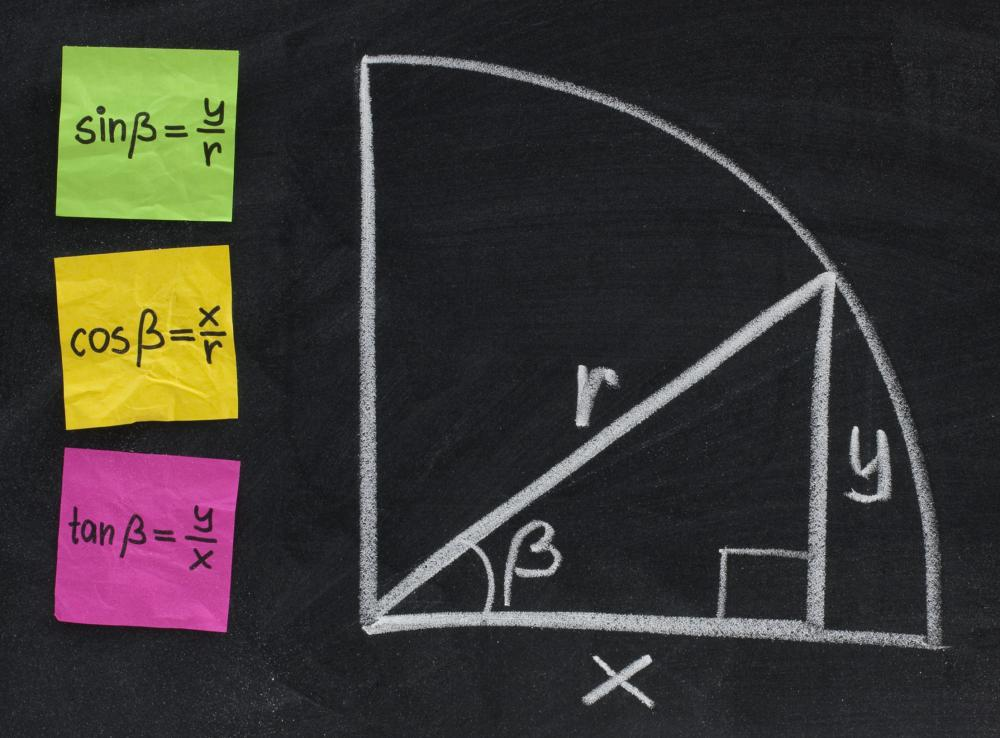# What Is Angular Distance?

Jerry Morrison

Angular distance is a measure of the apparent separation between two points from the perspective of the observer. Straight lines extended from each point to the observer intersect. The angle of intersection of these two lines is the angular distance, and is typically expressed in degrees or radians. In trigonometry, this angle can be used to calculate height and distances. Astronomers often use the angle to describe the apparent separation between celestial bodies without reference to their actual distance.Angular distance can be used to calculate height and distances in trigonometry.

A common trigonometry problem involves calculating the height of a building. The line of sight angular separation between the top and bottom of the building at a known distance is sufficient information to determine its height. Calculations involving angular distance are common in surveying and targeting. Instead of degrees or radians, the military often finds it useful to express targeting calculations in terms of angular mil. This is 1/6400 of the circumference of a circle, or more conveniently, the angular distance between two points separated by one meter at a range of 1000 meters.

In astronomy, there are two basic ways of describing the position of an object in the sky. One is by reference to a coordinate system, the other is by the object's position relative to another body. In the equatorial coordinate system, the Earth's poles and equator are projected into space as the celestial poles and celestial equator. The position of a body is described by its declination, degrees north or south of the celestial equator, and its hour angle. This is the angular distance along the celestial equator between the observer's location and the celestial meridian, a circle passing directly above the observer and through the celestial poles.

For the amateur, angular distance might be used to help locate an astronomical object in relationship to a known body, or just in noting an interesting feature. Often, all that is necessary is an outstretched hand. At arm's length, the tip of the little finger subtends about one degree of arc. Three middle fingers subtend about four degrees, and a closed fist about ten. The distance from little finger to thumb of an open hand covers about 18 degrees.

Frequently, the professional and more serious observer use a measurement similar to angular distance called angular diameter. This is the apparent size of an astronomical object as seen from Earth. These diameters are quite small and are usually measured in arcseconds, or 1/3600th of a degree. As with terrestrial measurement, if the distance to an object is known, its angular diameter can be used to calculate its true size.

## You might also Like